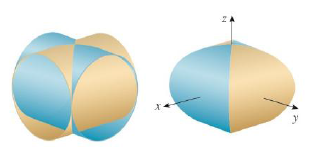Chapter 15.5, Problem 24E

Chapter
Section
Textbook Problem

The figure shows the surface created when the cylinder y2 + z2 = 1 intersects the cylinder x2 + z2 = 1. Find the area of this surface.To determine

To find: The area of the given surface.

Explanation

Formula used:

The surface area with equation z=f(x,y),(x,y)D , where fx and fy are continuous, is A(S)=D[fx(x,y)]2+[fy(x,y)]2+1dA .

Here, D is the given region.

Given:

The surface is the cylinder y2+z2=1 intersects another cylinder x2+z2=1 .

Calculation:

Solve the given equations,

y2+z2=x2+z2y2=x2y=±x2y=±x

Since the given surface is the cylinder of radius 1, x ranges from 0 to 1 in the first quadrant of the xy-plane. So, the total area is 8 times the surface area of the above mentioned region in the first quadrant.

The partial derivatives fx and fy are,

fx=2x21x2=xzfy=0

Then, by the formula mentioned above, the area of the surface is given by,

A(S)=D(xz)2+(0)2+1dA=Dx2z2+0+1dA=Dx2+z2z2dA=01xx11x2dydx

Integrate it with respect to y and apply the limit

Still sussing out bartleby?

Check out a sample textbook solution.

See a sample solution

The Solution to Your Study Problems

Bartleby provides explanations to thousands of textbook problems written by our experts, many with advanced degrees!

Get Started

Evaluate the integral. ln(1+x2)dx

Calculus (MindTap Course List)

In problems 33-36, write an inequality that discribes each interval or graph. 36.

Mathematical Applications for the Management, Life, and Social Sciences

Finding a Derivative In Exercises 9398, find F'(x). F(x)=0x2sin2d

Calculus: Early Transcendental Functions (MindTap Course List)

2 1 0 does not exist

Study Guide for Stewart's Multivariable Calculus, 8th

True or False: x2 + 6x + 2y = 1 defines y as a function of x.

Study Guide for Stewart's Single Variable Calculus: Early Transcendentals, 8th